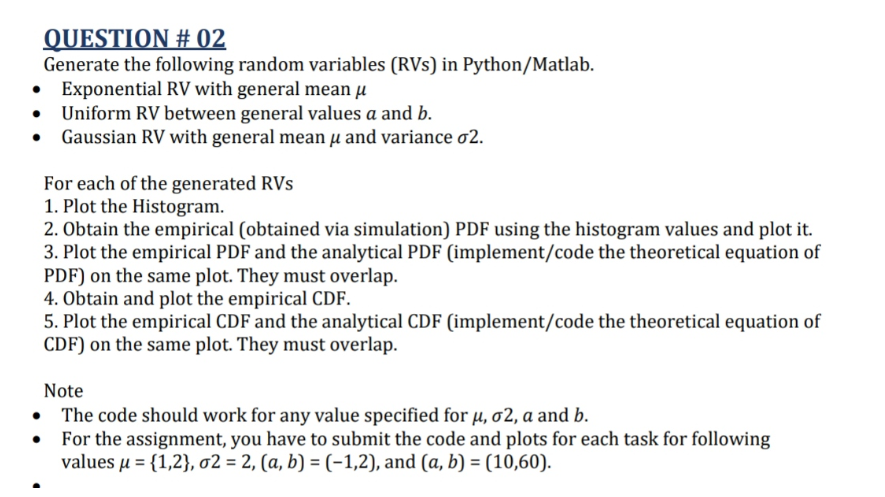Home / Expert Answers / Statistics and Probability / write-matlab-code-dont-copy-from-chatgpt-it-is-incorrect-question-02-generate-the-following-rand-pa894

# (Solved): write matlab code. dont copy from chatgpt it is incorrect QUESTION \# 02 Generate the following rand ...write matlab code. dont copy from chatgpt it is incorrect

QUESTION \# 02 Generate the following random variables (RVs) in Python/Matlab. - Exponential RV with general mean - Uniform RV between general values and . - Gaussian RV with general mean and variance . For each of the generated RVs 1. Plot the Histogram. 2. Obtain the empirical (obtained via simulation) PDF using the histogram values and plot it. 3. Plot the empirical PDF and the analytical PDF (implement/code the theoretical equation of PDF) on the same plot. They must overlap. 4. Obtain and plot the empirical CDF. 5. Plot the empirical CDF and the analytical CDF (implement/code the theoretical equation of on the same plot. They must overlap. Note - The code should work for any value specified for and . - For the assignment, you have to submit the code and plots for each task for following values , and .

We have an Answer from Expert